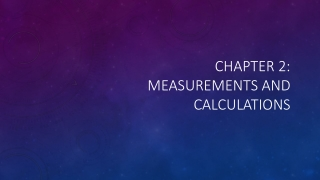DownloadDownload PresentationChapter 2: Measurements and Calculations

# Chapter 2: Measurements and Calculations

Download Presentation## Chapter 2: Measurements and Calculations

- - - - - - - - - - - - - - - - - - - - - - - - - - - E N D - - - - - - - - - - - - - - - - - - - - - - - - - - -
##### Presentation Transcript

1. Chapter 2:Measurements and Calculations

2. Scientific Method • Logical approach to solving problems by observing and collecting data, formulating hypotheses, testing hypotheses, and formulating theories that are supported by data. • Observing: the use of senses to obtain information • Qualitative vs. Quantitative • Examples? • System: Specific portion of matter in a given region of space that has been selected for study during an experiment or observation • Examples?

3. Hypothesis • Testable statement • If-then statements • Ex. If sunlight stimulates plant growth, then plants placed in direct sunlight will grow faster than those placed in the shade. • Need data from an experiment to support or refute the hypothesis (NOT prove or disprove) • Control vs. Variable

4. Theorizing • When data supports the hypothesis, scientists try to explain the phenomenon with a model • Model: an explanation of how phenomena occur and how data or events are located • Can be in picture/physical form but not always the case • Theory: broad generalization that explains a body of facts or phenomena • https://www.sciencenews.org/blog/context/top-10-revolutionary-scientific-theories

5. Plant Growth? • Design a lab using the scientific method that will explore what makes plants grow. • Use the lab report from yesterday as a starting point/reference. • Explain which type of research this would fall into and give evidence to support your claim.

6. Measurements • Quantity: something that has magnitude, size or amount • Quantity is not the same thing as a measurement • Ex. A teaspoon is a unit of measurement and volume is its quantity • Measurements require a unit • Measurements were not always standard • Ex. A foot was not always 12 inches

7. SI Measurements • Le Systeme International d’Unites • Abbreviated to SI • Adopted in 1960 by the General Conference on Weights and Measures • SI base units • Meter, kilogram, second, kelvin, mole, ampere, candela • Prefixes (ex. Mega, kilo, hecta, deka, deci, centi, milli, micro, nano, pico)

8. Derived Units

9. Conversion Factors • A ratio derived from the equality between two different units that can be used to convert from one unit to the other • Ex. Quarters in a dollar  How many quarters are in 5.25 dollars? • Dimensional Analysis: math technique that allows you to use units to solve problems involving measurements • Solve the problem above using both conversion factors to demonstrate how you choose the correct conversion factor.

10. Tips and Tricks • When there is not a number in the denominator, you assume 1. • When asking ”How do I begin the setup,” always start with what you know and identify where you want to go. This will help you pick the correct conversion factor. • ALWAYS DOUBLE CHECK YOUR UNITS . • Cancel out any units that appear both on the top and bottom of any conversion factors you use in a problem.

11. Accuracy VS Precision • Accuracy: the closeness of measurements to the correct or accepted value of the quantity measured • Close to the desired value • Precision: closeness of a set of measurements of the same quantity made in the same way • Close to one another but not necessarily close to the desired value

12. Percentage Error pg. 45

13. Density